# How to deal with EEG data ?

Tutorial

Collecting, filtering, slicing and understanding EEG data

Informations
• axel.thevenot@edu.devinci.fr
• 06 24 98 20 33
Dates
• Creation: 03/18/2020
• Update: 08/26/2020
axel_thevenotinnovationopenbci

Electroencephalogram (EEG) signal processing

Electroencephalography (EEG) is a method of brain imaging that measures electrical stimulation of the brain using electrodes mounted on the head. In this tutorial, I had a "Mark IV" Ultracortex electroencephalogram from OpenBCI that you can find here. Aware that this object is not available to everyone, I decided throughout this tutorial to simulate the signals emitted by the EEG from a text file found on their github.The EEG can be used for thought detection for example to know what color the user is thinking. But this is not the subject of this tutorial. We will see how to process these raw signals from an EEG (although some notions obviously apply to any type of signal). So we will see how to filter a signal, how to attenuate the noise contained in the signal and what the spectra of the signals are. Finally more specifically to brain waves we will see what are Delta, Theta, Alpha, Beta and Gamma waves and how to extract them.

Raw Data

On this file are simulated the data of 8 electrodes placed on the head according to the following diagram which represents the Ultracortex "Mark IV" flattened and seen from above. So we have the purple and grey electrodes on the forehead, the blue and green ones on the top of the skull, the yellow and orange ones behind the ears and finally the red and brown ones at the top of the neck.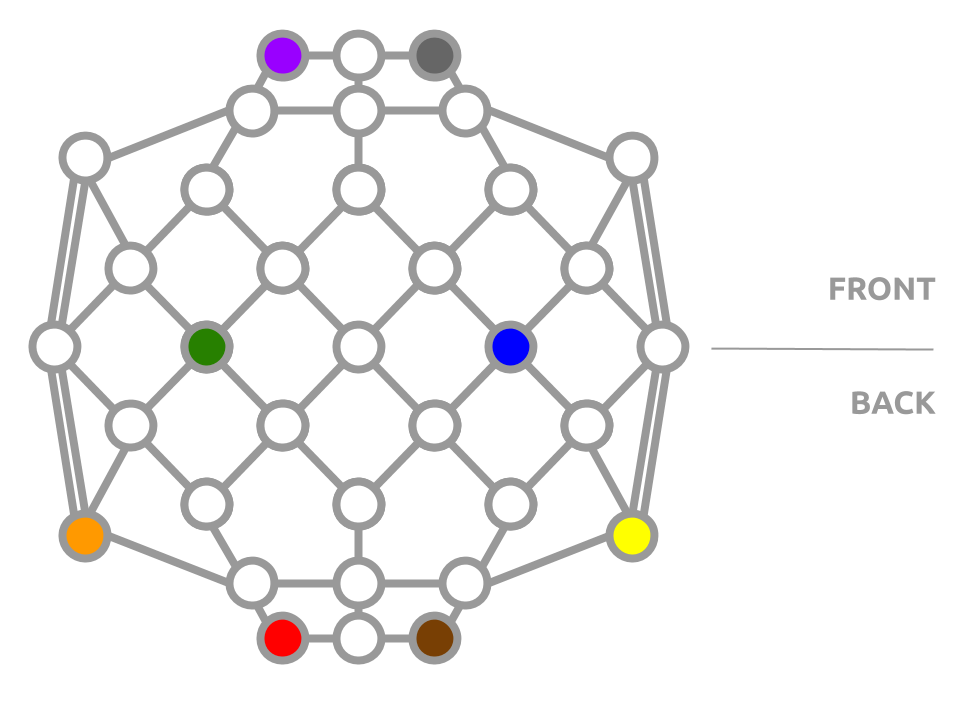We know then that we have 8 electrodes so 8 distinct signals (or sequence). Each electrode measures a potential difference. In the data set of the *.txt file, the potential differences measured (in mV) by the electrodes are evaluated 250 times per second. This file contains 22490 lines so we have the equivalent of 90 seconds of recording. Recording that we will use with a 3 seconds window to simulate the use of a real EEG.

```import time

import numpy as np
import matplotlib.pyplot as plt
from scipy import signal

plt.ion()

﻿class EEG:
def __init__(self, fs, time_window, colors, save_path):
"""
Initialize an EEG class with saved data
"""
# get all the saved sequences
self.sequences = np.genfromtxt(save_path, delimiter=',')
self.fs = fs
self.channels_color = colors
# compute de length of the window to return
self.len_window = time_window * self.fs
# get the length in time of the sequence
self.max_time = self.sequences.shape / self.fs

def get_sequences(self):
"""
Return a sequence by looping over the saved
sequences according to the current time
"""
# get the current time mod length in time to loop over the sequece
t = time.time() % self.max_time
# get the associated start index in this sequence
start_idx = int(t * self.fs)
# compute the stop index according of the window size asked
stop_idx = start_idx + self.len_window
return self.sequences[start_idx:stop_idx]
```

We can now read and display the data collected by the EEG in real time (simulated here).

```if __name__ == '__main__':
fs = 250 # in Hz
time_window = 3 # in seconds
colors = ['#8800ff', '#999999', # purple and grey
'#00ff00', '#0000ff', # green and blue
'#ff8800', '#ffff00', # orange and yellow
'#ff0000' ,'#884400'] # red and brown

# get a simulation of an eeg
eeg = EEG(fs, time_window, colors,  'eeg_data.txt')

fig_sig, ax_sig = plt.subplots(1, 1)
fig_sig.suptitle('Brain Signals')

while True:
sequences = eeg.get_sequences()

ax_sig.clear()
for i, c in enumerate(eeg.channels_color):
times = np.array(range(len(sequences[:, i])))
times = (times - np.max(times)) / eeg.fs

ax_sig.plot(times, sequences[:, i], c=c)
ax_sig.set_xlabel('Time (s)')
ax_sig.set_ylabel('Amplitude (mV)')

plt.pause(1e-7)
```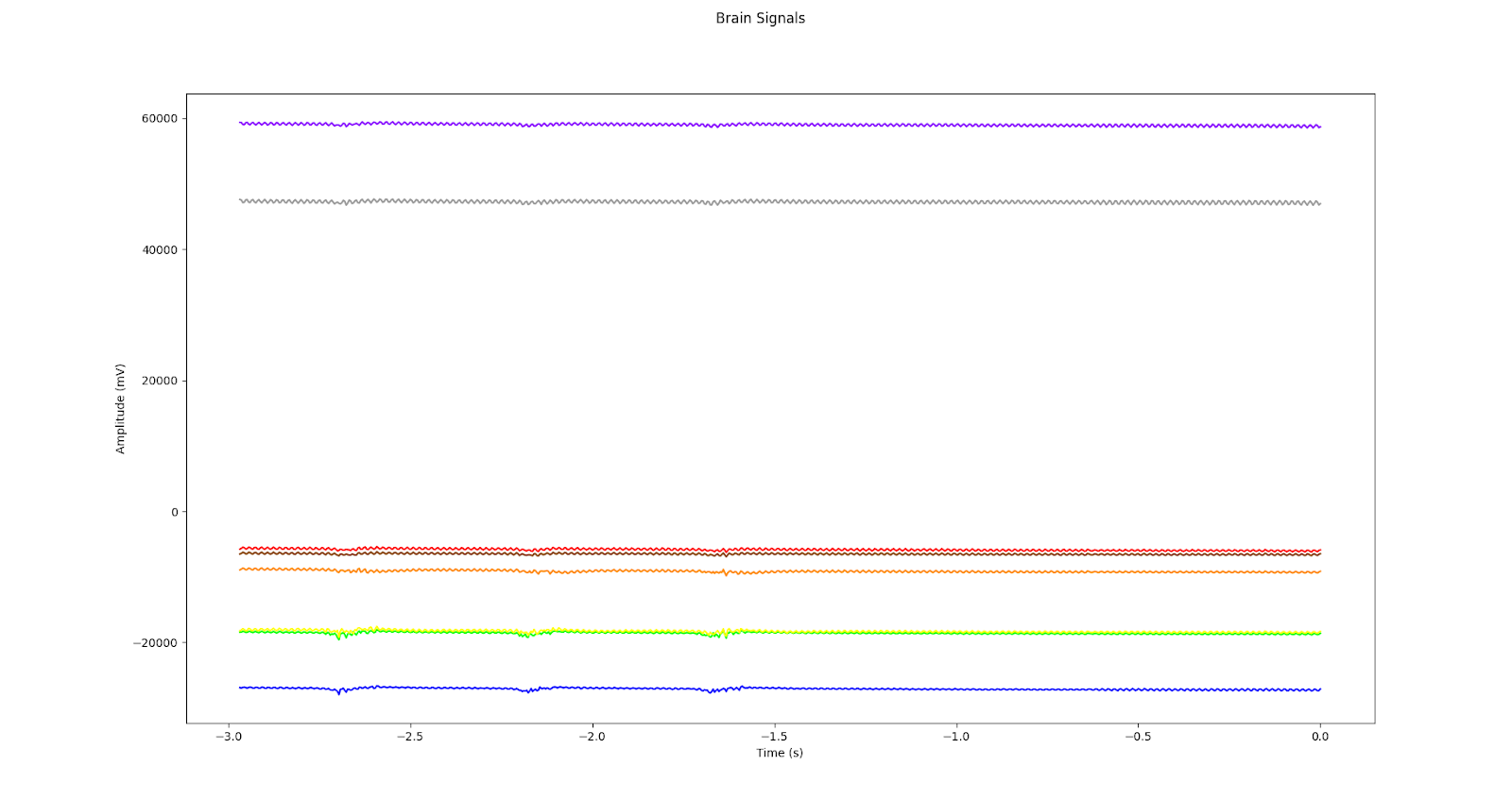Signal spectrum

If we were to tell a child what a signal is, we could simply say that it is waves moving. There is a time between each wave that is often referred as the T period. The wave also has a height that is called amplitude, which is called amplitude, and is often called A. So if we have waves of period T = 3s and height A = 2 then its associated signal is the curve below. We can clearly see that it repeats every 3 seconds and that it goes up/down at the maximum with an amplitude of 2 units.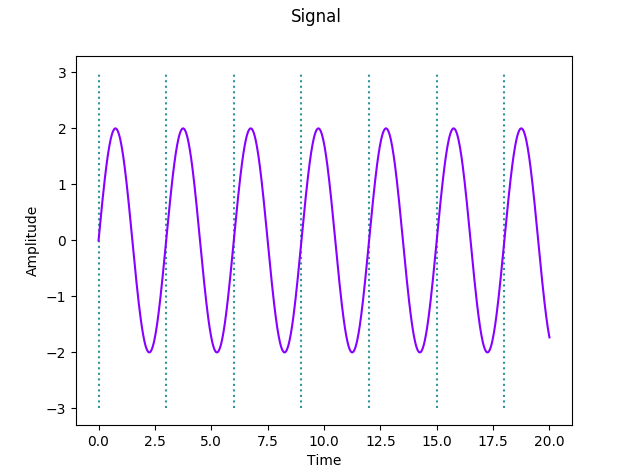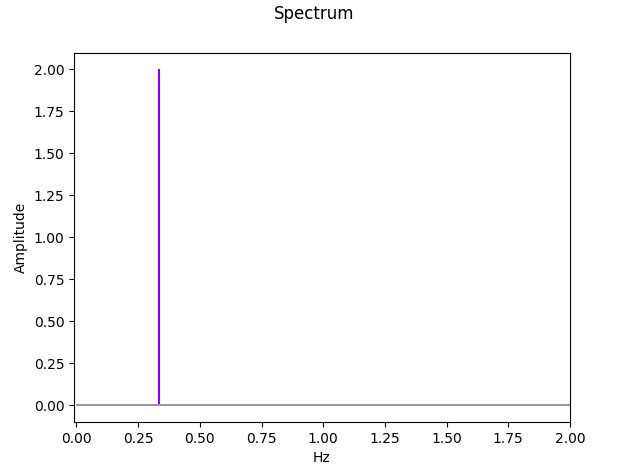What we call its spectrum is really only what we just said about the wave. On the spectrum of the signal we can directly see that the wave has an amplitude of 2 and that it repeats itself 0.33 times per second ( that is 1 time every period T=3s). We say that the wave has a frequency of 0.33 Hz. However we never deal with such simple signals having only one frequency of spectrum (a stick). For example if we take waves of period 3, 1 and 0.8 with amplitude 2, 5 and 4 respectively. Shown below, the signal is difficult to decompose with the eye, whereas by looking at its spectrum we easily have all the information of this signal.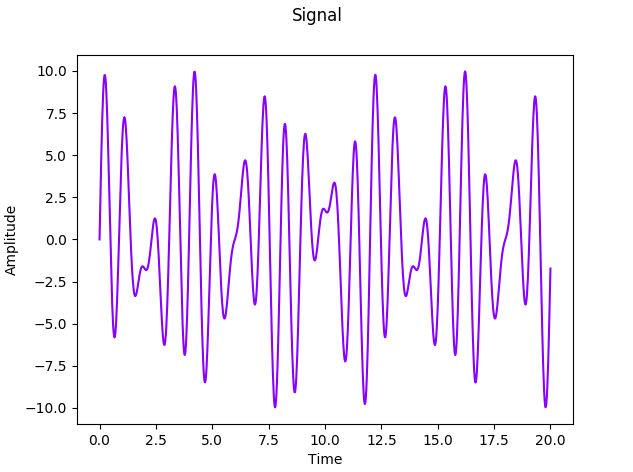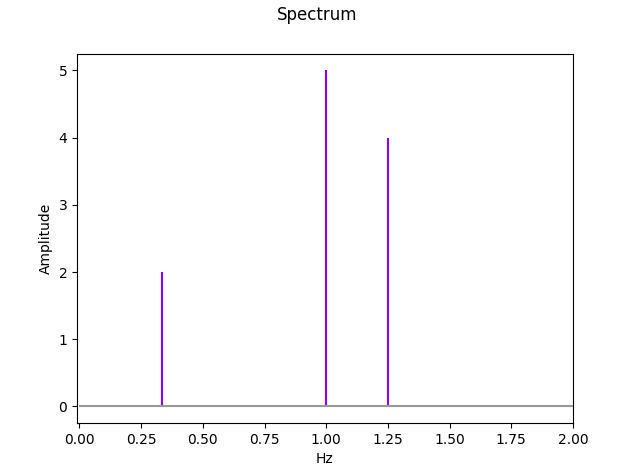We can then understand the interest of measuring the spectrum of a signal. It is nothing more than the description of all the waves that compose it. We can then use this same method for each of our signals coming from our EEG. Let us note that if the signal was centered in 4 for example, then we would have had a value of 4 for Hz = 0. (average of the signal)

The transition from the signal to its spectrum is called the Fourier Transformation.

Before implementing the function that will perform this transformation on our signals we still need to understand one last notion. The Nyquist frequency is the maximum frequency that a signal must contain in order to be able to describe it unambiguously when it is sampled. It is equal to half the sampling frequency.

To put it simply, a signal is the sum of waves that have a frequency. With our EEG we only get the values "only" 250 times per second. 250 is our sampling frequency. Nyquist's theorem tells us that if our signal does not include waves having a frequency lower than 125 Hz, then we will have an unambiguous description of this signal.

To better understand this notion, let's imagine that we have a signal with a frequency f=1 Hz. The GIF below shows us that if we take values below fs = 2 * f = 2 then we cannot be sure that our points describe our signal well. The proof is for fs = 2, we end up with a continuous signal.Now that all these notions are well assimilated we can then implement our method to apply the Fourier transformation.

```def fft(data, fs):
N = len(data) # number of observation
# get the frequencies according to Nyquist's Theorem
freq = np.linspace(0.0, 1.0 / (2.0 * fs **-1), N // 2)
# compute the Fourier's transformation
yi = np.fft.fft(data) / N
# extract the spectrum (if not, it will be a symetrical spectrum)
y = yi[range(int(N / 2))]
return freq, abs(y) # thhe spectrum is absolute
```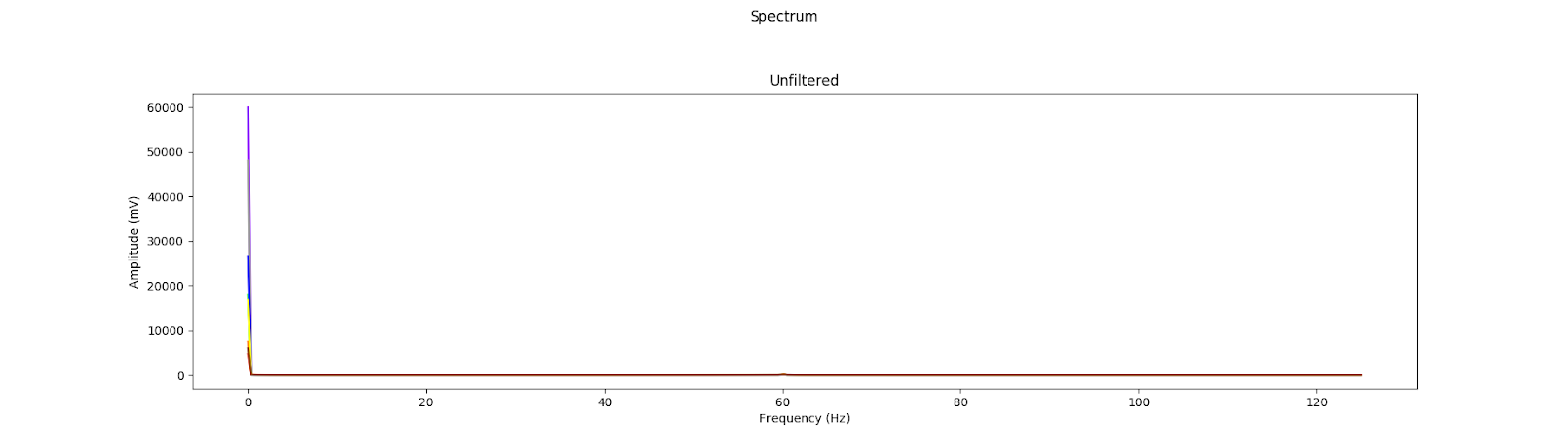And so apply this transformation to all the signals coming from our EEG. We can then notice that the average of these signals (In Hz = 0) is very high compared to the amplitudes of the other frequencies that make up our signals. Then we can in a readability concern, not to display the amplitude describing the average of our signal.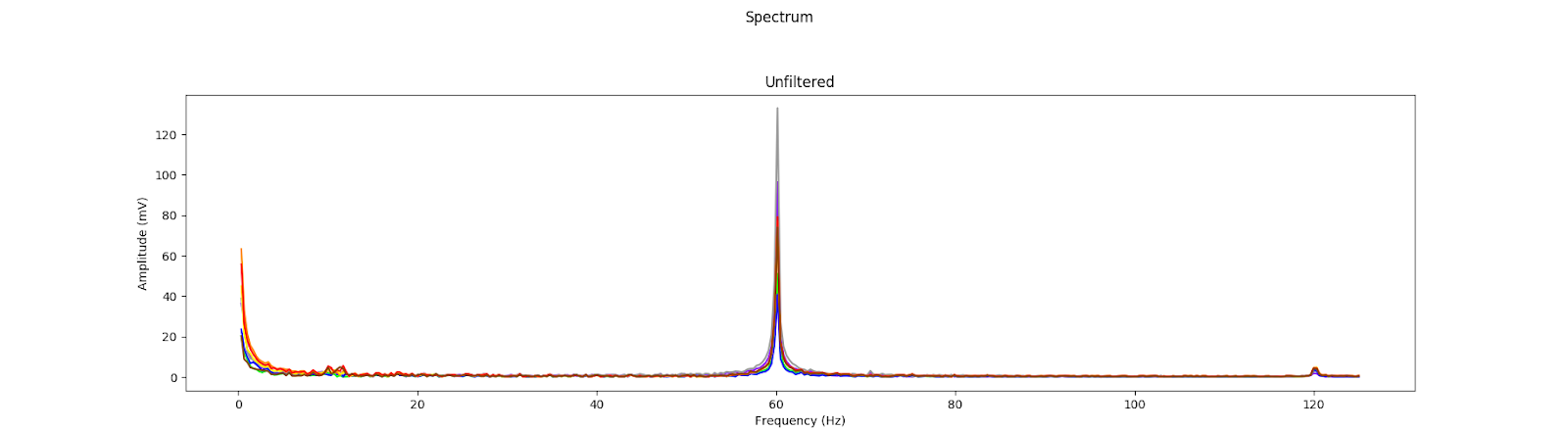We can then change the main as follows.

```if __name__ == '__main__':
fs = 250 # in Hz
time_window = 3 # in seconds
colors = ['#8800ff', '#999999', # purple and grey
'#00ff00', '#0000ff', # green and blue
'#ff8800', '#ffff00', # orange and yellow
'#ff0000' ,'#884400'] # red and brown

# get a simulation of an eeg
eeg = EEG(fs, time_window, colors,  'eeg_data.txt')

fig_sig, ax_sig = plt.subplots(1, 1)
fig_sig.suptitle('Brain Signals')

fig_spec, axs_spec = plt.subplots(2, 1)
fig_spec.suptitle('Spectrum')

while True:
sequences = eeg.get_sequences()

for ax in [ax_sig, *axs_spec]:
ax.clear()
for i, c in enumerate(eeg.channels_color):
times = np.array(range(len(sequences[:, i])))
times = (times - np.max(times)) / eeg.fs

ax_sig.plot(times, sequences[:, i], c=c)
ax_sig.set_xlabel('Time (s)')
ax_sig.set_ylabel('Amplitude (mV)')
# compute the spectrum
freq, spectrum = fft(sequences[:, i], eeg.fs)
freq, spectrum = freq[1:], spectrum[1:] # remove the constant
axs_spec.plot(freq, spectrum, c=c)
axs_spec.set_title('Unfiltered')
axs_spec.set_xlabel('Frequency (Hz)')
axs_spec.set_ylabel('Amplitude (mV)')

plt.pause(1e-7)
```

Filter a signal

As can be seen in the spectrum of our signal, there is a strong amplitude for frequencies around 60Hz. These are not useful frequencies, on the contrary, they are actually electrode noise. A place has been left on the last plot to be able, at the end of this part, to display the spectrum of the filtered signal.

As said, frequencies around 60Hz are not data to be taken into consideration since they are only noise. We can therefore pre-process the signal to remove this noise, or at least attenuate it. This processing is done using filters.There are several types of filters. The four most common filters are low-pass, high-pass, band-pass and band-stop filters. The low-pass filter, as its name implies, allows frequencies below a certain threshold to pass through. For example, a 60Hz low-pass filter lets frequencies pass up to 60Hz and then lets nothing pass beyond that. The opposite phenomenon is observed for the high-pass filter.

Finally, a bandpass filter will pass frequencies between two given frequencies. The bandpass filter above will pass all frequencies between 30Hz and 90Hz. The reverse is true for a band-stop filter.

We can notice that for all filters, there is a transition between the frequencies that pass and those that do not pass. This transition depends on the order of the filter. The higher the filter order, the shorter the transition will be.```def butter_notch(cut, fs, order=9):
b, a = signal.iirnotch(cut, order, fs)
return b, a

def butter_lowpass(cut, fs, order=9):
nyq = 0.5 * fs
cut = cut / nyq
b, a = signal.butter(order, cut, btype='high')
return b, a

def butter_highpass(cut, fs, order=9):
nyq = 0.5 * fs
cut = cut / nyq
b, a = signal.butter(order, cut, btype='high')
return b, a

def butter_bandpass(lowcut, highcut, fs, order=9):
nyq = 0.5 * fs
low = lowcut / nyq
high = highcut / nyq
b, a = signal.butter(order, [low, high], btype='band')
return b, a

def butter_bandstop(lowcut, highcut, fs, order=9):
nyq = 0.5 * fs
low = lowcut / nyq
high = highcut / nyq
b, a = signal.butter(order, [low, high], btype='bandstop')
return b, a
```

Let's get back to the subject. Now that we understand what a filter is for, let's see what we want to do with it. To remove noise at 60Hz, the adequate filter among the four presented above is the band-stop filter. For example with a band-stop filter around 60Hz. But we are not going to use this filter. The Notch filter is more suitable. It allows much better to filter a given frequency without filtering too much the others.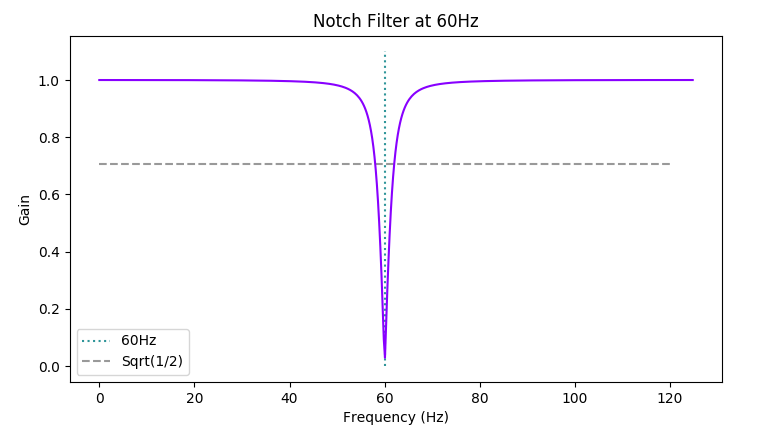So we can apply this filter to our signal to remove the noise we have. As a reminder, the noise is at 60Hz. And to smooth the spectrum afterwards, we will use a function to compute a bilateral exponential moving average.

```def billateral_ema(data, alpha):
forward = np.zeros_like(data)
forward = data
for i in range(len(data) - 1):
forward[i + 1] = data [i + 1] * alpha + (1 - alpha) * forward[i]
backward = np.zeros_like(data)
backward[-1] = data[-1]
for i in range(len(data) - 1, 2, -1):
backward[i - 1] = data[i - 1] * alpha + (1 - alpha) * backward[i]
return (forward + backward) / 2
```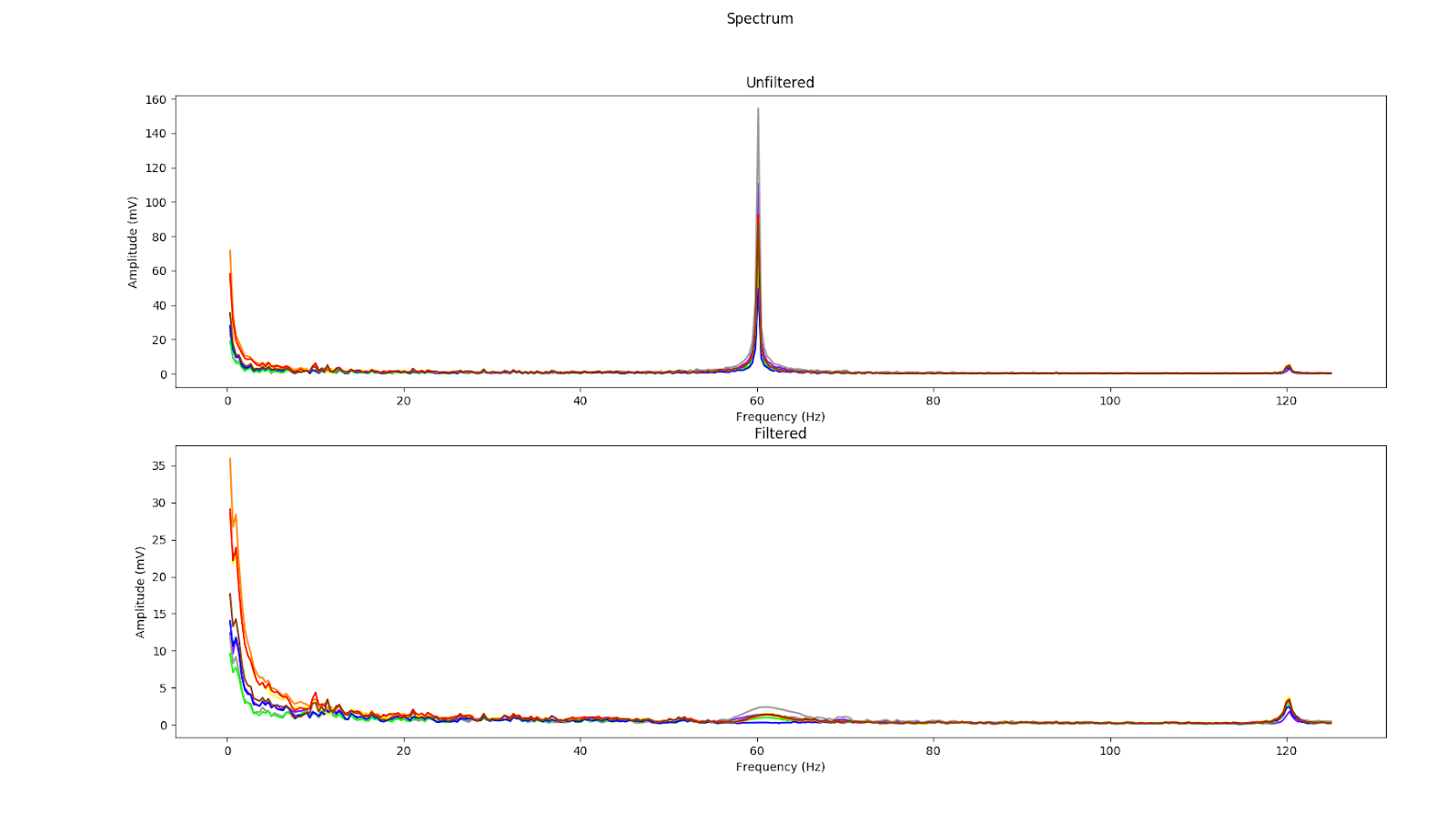We can then rewrite our main part.

```if __name__ == '__main__':
fs = 250 # in Hz
time_window = 3 # in seconds
colors = ['#8800ff', '#999999', # purple and grey
'#00ff00', '#0000ff', # green and blue
'#ff8800', '#ffff00', # orange and yellow
'#ff0000' ,'#884400'] # red and brown

# get a simulation of an eeg
eeg = EEG(fs, time_window, colors,  'eeg_data.txt')

fig_sig, ax_sig = plt.subplots(1, 1)
fig_sig.suptitle('Brain Signals')

fig_spec, axs_spec = plt.subplots(2, 1)
fig_spec.suptitle('Spectrum')

while True:
sequences = eeg.get_sequences()

for ax in [ax_sig, *axs_spec]:
ax.clear()
for i, c in enumerate(eeg.channels_color):
times = np.array(range(len(sequences[:, i])))
times = (times - np.max(times)) / eeg.fs

ax_sig.plot(times, sequences[:, i], c=c)
ax_sig.set_xlabel('Time (s)')
ax_sig.set_ylabel('Amplitude (mV)')
# compute the spectrum
freq, spectrum = fft(sequences[:, i], eeg.fs)
freq, spectrum = freq[1:], spectrum[1:] # remove the constant
axs_spec.plot(freq, spectrum, c=c)
axs_spec.set_title('Unfiltered')
axs_spec.set_xlabel('Frequency (Hz)')
axs_spec.set_ylabel('Amplitude (mV)')

b, a = butter_notch(60, eeg.fs)
seq = signal.filtfilt(b, a, sequences[:, i])
freq, spectrum = fft(seq, eeg.fs)
freq, spectrum = freq[1:], spectrum[1:] # remove the constant
spectrum = billateral_ema(spectrum, alpha=0.5)
axs_spec.plot(freq, spectrum, c=c)
axs_spec.set_title('Filtered')
axs_spec.set_xlabel('Frequency (Hz)')
axs_spec.set_ylabel('Amplitude (mV)')

plt.pause(1e-7)
```

The different brain waves

Now that we have our preprocess done for our EEG signals, all we have to do is look at the different brain waves. The different brain waves are generally categorized into 5 distinct segments. We note the Delta, Theta, Alpha Beta and Gamma waves. Depending on the source, these categories correspond to different frequency bands.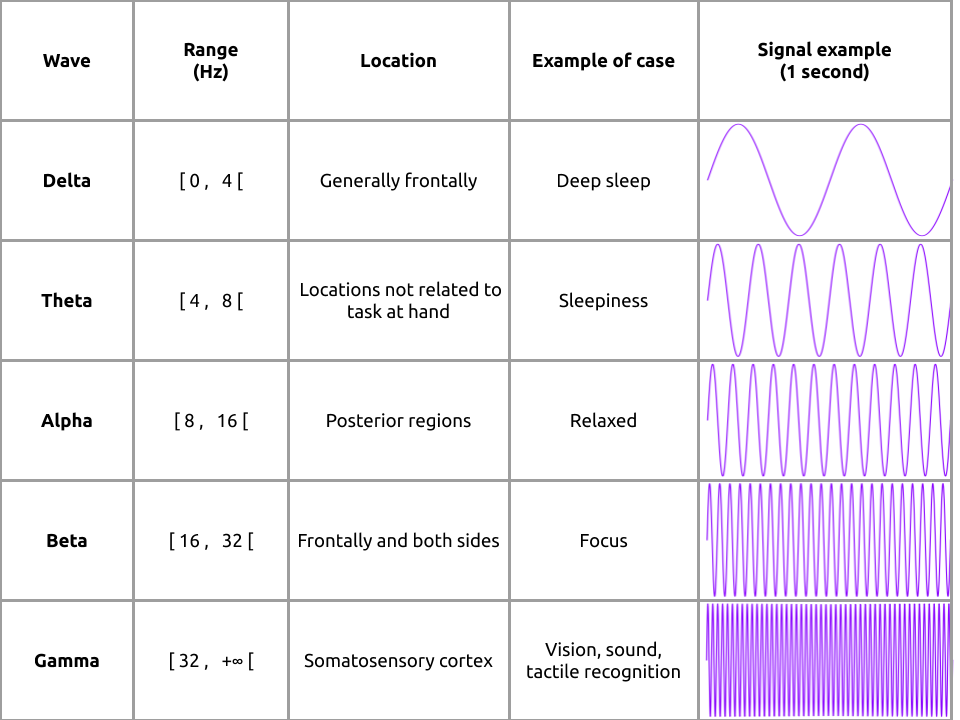To filter these different waves we could use band-pass filters but we already have all the information available and this would cause us to lose information. So we just have to cut our spectrum according to these different waves.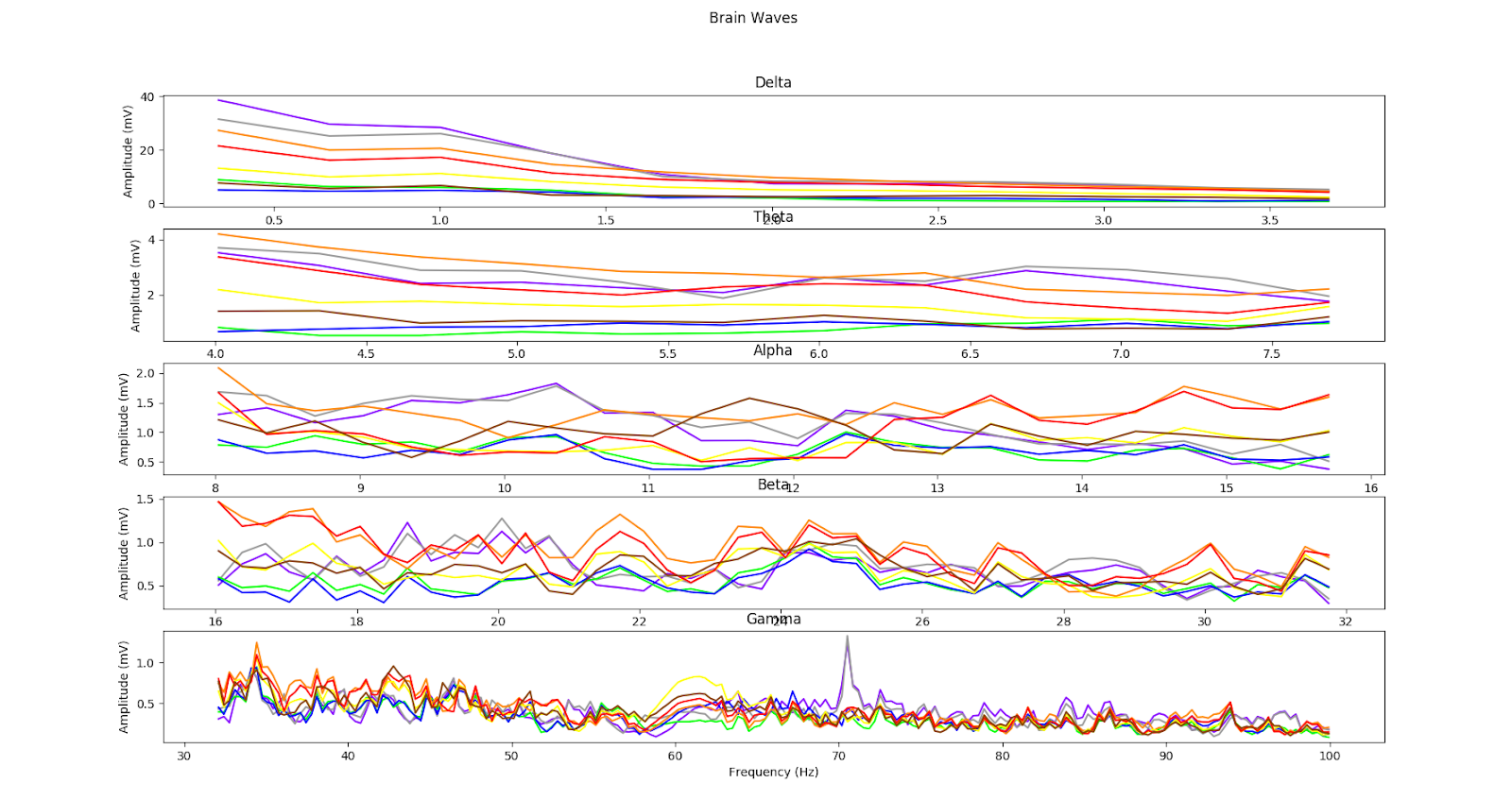```if __name__ == '__main__':
fs = 250 # in Hz
time_window = 3 # in seconds
colors = ['#8800ff', '#999999', # purple and grey
'#00ff00', '#0000ff', # green and blue
'#ff8800', '#ffff00', # orange and yellow
'#ff0000' ,'#884400'] # red and brown

# get a simulation of an eeg
eeg = EEG(fs, time_window, colors,  'eeg_data.txt')

fig_sig, ax_sig = plt.subplots(1, 1)
fig_sig.suptitle('Brain Signals')

fig_spec, axs_spec = plt.subplots(2, 1)
fig_spec.suptitle('Spectrum')

waves = {
'Delta': (0, [0, 4]),
'Theta': (1, [4, 8]),
'Alpha': (2, [8, 16]),
'Beta': (3, [16, 32]),
'Gamma': (4, [32, 100])
}

fig_waves, axs_waves = plt.subplots(len(waves), 1)
fig_waves.suptitle('Brain Waves')

while True:
sequences = eeg.get_sequences()

for ax in [ax_sig, *axs_spec, *axs_waves]:
ax.clear()
# for ax in [*axs_spec, ax_sig, *axs_waves]:
#     ax.clear()
# for ax in [*axs_spec, ax_sig, *axs_waves]:
#     ax.clear()
for i, c in enumerate(eeg.channels_color):
times = np.array(range(len(sequences[:, i])))
times = (times - np.max(times)) / eeg.fs

ax_sig.plot(times, sequences[:, i], c=c)
ax_sig.set_xlabel('Time (s)')
ax_sig.set_ylabel('Amplitude (mV)')
# compute the spectrum
freq, spectrum = fft(sequences[:, i], eeg.fs)
freq, spectrum = freq[1:], spectrum[1:] # remove the constant
axs_spec.plot(freq, spectrum, c=c)
axs_spec.set_title('Unfiltered')
axs_spec.set_xlabel('Frequency (Hz)')
axs_spec.set_ylabel('Amplitude (mV)')

b, a = butter_notch(60, eeg.fs)
seq = signal.filtfilt(b, a, sequences[:, i])
freq, spectrum = fft(seq, eeg.fs)
freq, spectrum = freq[1:], spectrum[1:] # remove the constant
spectrum = billateral_ema(spectrum, alpha=0.5)
axs_spec.plot(freq, spectrum, c=c)
axs_spec.set_title('Filtered')
axs_spec.set_xlabel('Frequency (Hz)')
axs_spec.set_ylabel('Amplitude (mV)')

step_freq = np.max(freq) / len(freq)
for wave_name, (ax_idx, [mini, maxi]) in waves.items():
mini_idx = int(mini / step_freq)
maxi_idx = int(maxi / step_freq)
axs_waves[ax_idx].plot(freq[mini_idx:maxi_idx], spectrum[mini_idx:maxi_idx], c=c)
axs_waves[ax_idx].set_title(wave_name)
axs_waves[ax_idx].set_xlabel('Frequency (Hz)')
axs_waves[ax_idx].set_ylabel('Amplitude (mV)')
plt.pause(1e-7)

```

Now we have all the keys to easily create the GIF you saw at the beginning of this tutorial. It represents the channels's spectrum according to the lasts time it has been calculated.

```import cv2
from imutils import rotate

def _hex2tuple(value, normalize=True):
# get the hexadecimal value
value = value.lstrip('#')
# get it length
lv = len(value)
# get the associated color in 0 to 255 base
color = np.array([int(value[i:i + lv // 3], 16) for i in range(0, lv, lv // 3)])
# normalize it if needed
if normalize:
color = tuple(color / 255)
return color

def get_img(data, eeg):
F, T, E, C = (*data.shape, 3)
imgs = [np.zeros((F, T, C)) for _ in range(E)]
for e, img in enumerate(imgs):
for c, val in enumerate(_hex2tuple(eeg.channels_color[e])[::-1]):
img[..., c] = val * data[..., e]
return np.hstack(imgs)

if __name__ == '__main__':
fs = 250 # in Hz
time_window = 3 # in seconds
colors = ['#8800ff', '#999999', # purple and grey
'#00ff00', '#0000ff', # green and blue
'#ff8800', '#ffff00', # orange and yellow
'#ff0000' ,'#884400'] # red and brown

# get a simulation of an eeg
eeg = EEG(fs, time_window, colors,  'eeg_data.txt')
# data memory
img_len = 50
data = np.zeros((eeg.len_window // 2,  img_len, len(eeg.channels_color)))

while True:
# sequences = np.array(get_data_to_dict(url))
sequences = eeg.get_sequences()
if sequences is None:
print('No data received')
continue

# roll on first axis
data = np.roll(data, 1, axis=1)

for i, c in enumerate(eeg.channels_color):

b, a = butter_notch(60, eeg.fs)
seq = signal.filtfilt(b, a, sequences[:, i])
freq, spectrum = fft(seq, eeg.fs)
spectrum = np.log(1 + spectrum)
data[:, 0, i] = spectrum

norm = data
img = get_img(norm, eeg)
img = rotate(img, 90)
img = cv2.flip(img, 0)
cv2.imshow('spectrum img', cv2.resize(img, (750, 750)))
if cv2.waitKey(1) & 0xff == ord('q'):
break
```